# How to add Drag fields with no identifier

In our worksheets we can make the students choose between several options where only one of them is correct. The correct one should be dragged to the Drop field, while the rest should not be dragged, as in the following example: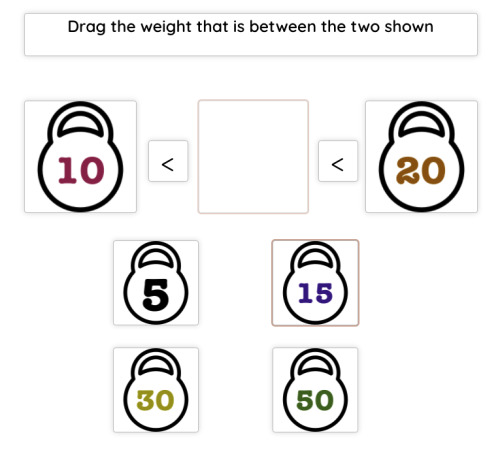To set up a worksheet like this, first add the Drop field with the identifier of your choice. Then add the different Drop fields with the options that will be offered to the student. In the correct option we will set the same identifier as in the Drop field. In the rest of the fields, which are not correct but we want the student to be able to drag so that he does not know which of them is correct, we will mark the option This drag doesn't have any related drop.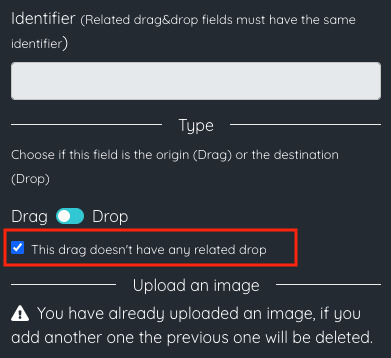This way, we don't have to set the identifier or which field it is related to.

When the student finishes the worksheet, only if he drags the field we have marked with identifier the full grade will be given. In case he drags other fields, the final grade will be calculated taking into account those fields and adjusting the final score accordingly.

Let's see it with some examples. We start with the two simplest examples: if you drag the correct field, so the final score will be a 10, or if you drag an incorrect field, so the final score will be a 0.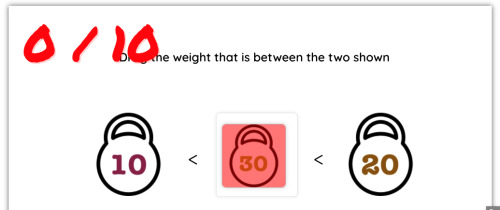There is also the possibility for students to drag more than one field at the same time. In that case, the final grade is adjusted according to the fields that have been dragged and the score of each field. As the final grade is calculated by the formula correct points / total points the correct fields will be added to both numerator and denominator, while the incorrect ones will be added only to the denominator. Let's also look at some examples.

If the student drags both a correct and an incorrect field (both with a score of 1), the formula will be calculated as 1 / (1 + 1) and, therefore, the final grade will be a 5.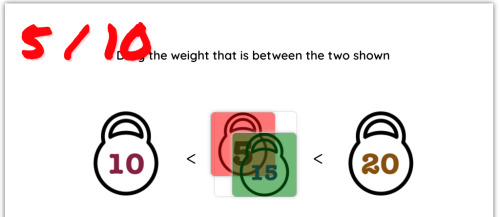If the student drags all the fields, the formula will be calculated as 1 / (1 + 1 + 1 + 1 + 1) and the final grade will be 2.5.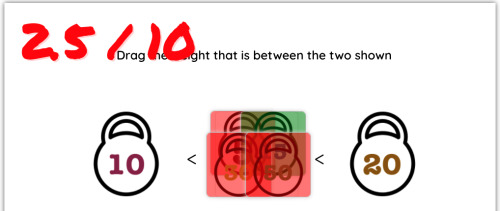We can make it even more complicated by changing the score of the different fields, increasing the score of incorrect questions to discourage random answers. We only have to take into account the formula indicated for the calculation of the final grade.